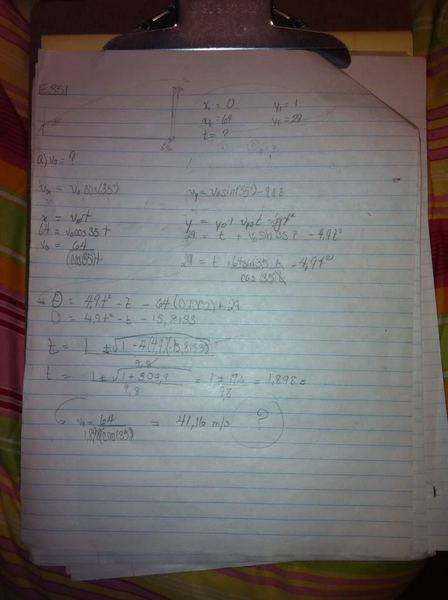# BASIC physics question: baseball going at Vo

• MissJewels
In summary: Start with:v_x = v_0\cos(\theta)v_y = v_0\sin(\theta) - gtWhat are the equations for the height and range as a function of time? Set height at 29 m and at horizontal range 64 m. Plug the values for x, y into these equations. From that you should be able to determine v_0.AMFrom the first equation:t = 64/v_0\cos{(35)}Substitute that value for t into the second equation:y = y_0 + v_{0}\sin{(35)}t

## Homework Statement

A baseball is hit at a height of 1m with an initial speed of vo module at an angle of projection of 35o from the horizontal. She spent just over an obstacle of 29m high at a horizontal distance of 64m.
Find
a) vo
b) the time it takes to reach the OBSTACLES
c) its speed at the obstacle.

## Homework Equations

Xi= 0
Xf= 64
Yi= 1
Yf= 29

Equations: Yf= Vosin(35) -9,8t

## The Attempt at a Solution

Not too sure how to go about with this one. I end up with two variables in the equations i use. help!

MissJewels said:

## Homework Statement

A baseball is hit at a height of 1m with an initial speed of vo module at an angle of projection of 35o from the horizontal. She spent just over an obstacle of 29m high at a horizontal distance of 64m.
Find
a) vo
b) the time it takes to reach the OBSTACLES
c) its speed at the obstacle.

$v_x = v_0\cos(\theta)$

$v_y = v_0\sin(\theta) - gt$

What are the equations for the height and range as a function of time?

Set height at 29 m and at horizontal range 64 m. Plug the values for x, y into these equations. From that you should be able to determine v_0.

AM

Andrew Mason said:

$v_x = v_0\cos(\theta)$

$v_y = v_0\sin(\theta) - gt$

What are the equations for the height and range as a function of time?

Set height at 29 m and at horizontal range 64 m. Plug the values for x, y into these equations. From that you should be able to determine v_0.

AM

Sorry it took a while, here's what I did... something's off...
just part a)From the first equation:

$t = 64/v_0\cos{(35)}$

Substitute that value for t into the second equation:

$y = y_0 + v_{0}\sin{(35)}t - \frac{1}{2}gt^2$

It gets a little hairy with a quadratic equation but I think it is a little easier to solve for v0 than for t.

AM